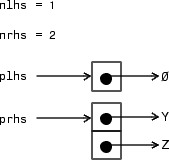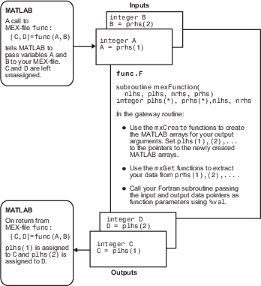## Data Flow in Fortran MEX Files

### Showing Data Input and Output

Suppose that your MEX file `myFunction` has two input arguments and one output argument. The MATLAB® syntax is `[X] = myFunction(Y, Z)`. To call `myFunction` from MATLAB, type:

`X = myFunction(Y, Z);`

The MATLAB interpreter calls `mexFunction`, the gateway routine to `myFunction`, with these arguments:Your input is `prhs`, a two-element array (`nrhs = 2`). The first element is a pointer to an `mxArray` named `Y` and the second element is a pointer to an `mxArray` named `Z`.

Your output is `plhs`, a one-element array (`nlhs = 1`) where the single element is a `null` pointer. The parameter `plhs` points at nothing because the output `X` is not created until the subroutine executes.

The gateway routine creates the output array and sets a pointer to it in `plhs`. If the routine does not assign a value to `plhs` but you assign an output value to the function when you call it, MATLAB generates an error.

Note

It is possible to return an output value even if ```nlhs = 0```, which corresponds to returning the result in the `ans` variable.

### Gateway Routine Data Flow Diagram

This MEX Cycle diagram shows how inputs enter a MEX file, what functions the gateway routine performs, and how outputs return to MATLAB.

In this example, the syntax of the MEX file `func` is `[C, D] = func(A,B)`. In the figure, a call to `func` tells MATLAB to pass variables `A` and `B` to your MEX file. `C` and `D` are left unassigned.

The gateway routine uses the `mxCreate*` functions to create the MATLAB arrays for your output arguments. It sets `plhs` and `plhs` to the pointers to the newly created MATLAB arrays. It uses the `mxGet*` functions to extract your data from your input arguments `prhs` and `prhs`. Finally, it calls your computational routine, passing the input and output data pointers as function parameters.

MATLAB assigns `plhs` to `C` and `plhs` to `D`.

Fortran MEX Cycle